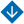A Parametric Lyapunov Function for Discrete-Time Control Systems with Bounded Exogenous Disturbances: Analysis
•About Advisory & Editorial Boards Aims & Scope Publication Ethics Review Process Archive Contact Information

#A Parametric Lyapunov Function for Discrete-Time Control Systems with Bounded Exogenous Disturbances: Analysis

Khlebnikov, M.V. and Kvinto, Ya.I. A Parametric Lyapunov Function for Discrete-Time Control Systems with Bounded Exogenous Disturbances: Analysis
Abstract. This paper considers a linear discrete-time dynamic system subjected to arbitrary bounded exogenous disturbances described by a matrix from a convex affine family. A simple approach to designing a parametric quadratic Lyapunov function for this system is proposed. It involves linear matrix inequalities and a fruitful technique to separate the system matrix and the Lyapunov function matrix in the matrix inequality expressing a stability condition of the system. Being well known, this technique, however, has not been previously applied to dynamic systems with nonrandom bounded exogenous disturbances. According to the numerical simulations, the parametric quadratic Lyapunov function-based approach yields appreciably less conservative results for the class of systems under consideration than the common quadratic Lyapunov function-based one.

Keywords: dynamic system, linear discrete-time system, parametric quadratic Lyapunov function, common quadratic Lyapunov function, bounded exogenous disturbances, robustness, linear matrix inequalities, analysis problem, conservatism, structured matrix uncertainty.
PDF (English)